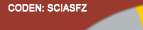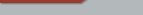| Home  | About ScienceAsia  | Publication charge  | Advertise with us  | Subscription for printed version  | Contact usEditorial Board Journal Policy Instructions for Authors Online submission Author Login Reviewer Login Volume 49 Number 2 Volume 49 Number 1 Volume 48 Number 6 Volume 48 Number 5 Volume 48 Number 4 Volume 48 Number 3 Earlier issues
 Volume 49 Number 3Research articles

ScienceAsia 49 (2023): 369-376 |doi: 10.2306/scienceasia1513-1874.2023.002

# On existence of meromorphic solutions for nonlinear q-difference equation

## Changwen Penga,*, Huawei Huangb, Lei Taob,c

ABSTRACT:     : In this paper, we mainly consider the existence of meromorphic solutions of nonlinear q-difference equation of type f (qz) + f (z/q) = P(z, f (z)) Q(z, f (z)), where the right-hand side is irreducible, P(z, f (z)) and Q(z, f (z)) are polynomials in f with rational coefficients, and q is a nonzero complex constant. We obtain that such equation has no transcendental meromorphic solution when |q| = 1 and m = degf (P)?degf (Q) > 1. And we investigate the growth of transcendental meromorphic solutions of nonlinear q-difference equation and find lower bounds for their characteristic functions for transcendental meromorphic solutions of such equation for the case |q| ?= 1.